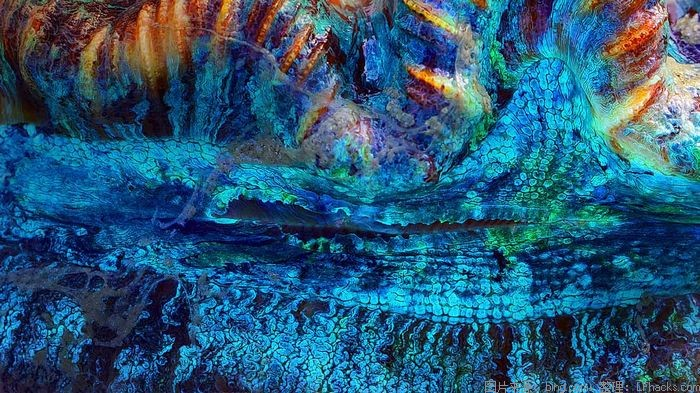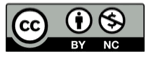# 喷泉取水问题（工程问题和相遇问题）

2021-04-22## 题面## 题型总结• 完成一个项目
• 运走一堆沙子
• 填满一个池子
• 花完一笔款项

## 消费速率

• 消费者是杯子时，消费速率就是杯子每天装的水
• 消费者是工程队时，消费速率就是工程队每天干的活
• 消费者是运动者时，消费速率就是每天走的路程
• 消费者是物品时，消费速率就是每件物品的钱

## 上面的题解

• 第一个泉：每天填满杯子容积
• 第二个泉：每天填满杯子容积的 $\frac{1}{2}$
• 第三个泉：每天填满杯子容积的 $\frac{1}{3}$
• 第四个泉：每天填满杯子容积的 $\frac{1}{4}$

$$t = \frac{1} {1+ \frac{1}{2} + \frac{1}{3} + \frac{1}{4}} = \frac{12}{25}天$$

## 精彩评论